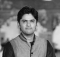## Deleting occurrences of an element if it occurs more than n times using JavaScriptPublished on 21-Apr-2021 10:11:17
ProblemWe are required to write a JavaScript function that takes in an array of numbers as the first argument and a number as the second argument.Our function should delete elements from the array so that the resulting array contains no element appearing for more than the specified number (second argument).ExampleFollowing ... Read More

## Sorting 2-D array of strings and finding the diagonal element using JavaScriptPublished on 21-Apr-2021 10:10:32
ProblemWe are required to write a JavaScript function that takes in an array of n strings. And each string in the array consists of exactly n characters.Our function should first sort the array in alphabetical order. And then return the string formed by the characters present at the principal diagonal ... Read More

## Finding the sum of all common elements within arrays using JavaScriptPublished on 21-Apr-2021 10:09:48
ProblemWe are required to write a JavaScript function that takes in three arrays of numbers. Our function should return the sum of all those numbers that are common in all three arrays.ExampleFollowing is the code − Live Democonst arr1 = [4, 4, 5, 8, 3]; const arr2 = [7, 3, 7, ... Read More

## Greatest number divisible by n within a bound in JavaScriptPublished on 20-Apr-2021 13:19:40
ProblemWe are required to write a JavaScript function that takes in a number n and bound number b.Our function should find the largest integer num, such that −num is divisible by divisornum is less than or equal to boundnum is greater than 0.ExampleFollowing is the code − Live Democonst n = ... Read More

## Finding the sum of minimum value in each row of a 2-D array using JavaScriptPublished on 20-Apr-2021 13:19:01
ProblemWe are required to write a JavaScript function that takes in a 2-D array of numbers. Our function should pick the smallest number from each row of the 2-D array and then finally return the sum of those smallest numbers.ExampleFollowing is the code − Live Democonst arr = [    [2, ... Read More

## Finding the only out of sequence number from an array using JavaScriptPublished on 20-Apr-2021 13:18:21
ProblemWe are required to write a JavaScript function that takes in an array of numbers. The array is sorted in ascending / increasing order and only one element in the array is out of order.Our function should find and return that element.ExampleFollowing is the code −const arr = [1, 2, ... Read More

## Counting the number of letters that occupy their positions in the alphabets for array of strings using JavaScriptPublished on 20-Apr-2021 13:17:47
ProblemWe are required to write a JavaScript function that takes in an array of strings of english lowercase alphabets.Our function should map the input array to an array whose corresponding elements are the count of the number of characters that had the same 1-based index in the index as their ... Read More

## Breaking a string into chunks of defined length and removing spaces using JavaScriptPublished on 20-Apr-2021 13:17:07
ProblemWe are required to write a JavaScript function that takes in a string sentence that might contain spaces as the first argument and a number as the second argument.Our function should first remove all the spaces from the string and then break the string into a number of chunks specified ... Read More

## Finding the count of numbers divisible by a number within a range using JavaScriptPublished on 20-Apr-2021 13:15:12
ProblemWe are required to write a JavaScript function that takes in a range of two integers as the first argument and a number as the second argument.Our function should find all the numbers divisible by the input number in the specified range and return their count.ExampleFollowing is the code − Live ... Read More

## Constructing an array of first n multiples of an input number in JavaScriptPublished on 20-Apr-2021 13:14:33
ProblemWe are required to write a JavaScript function that takes in two numbers, let say m and n.Our function should construct and return an array of first n natural multiples of m.ExampleFollowing is the code − Live Democonst m = 6; const n = 14; const firstNMultiple = (m = 1, ... Read More
Advertisements